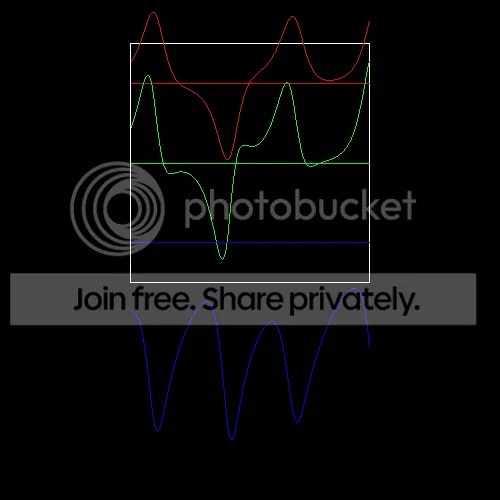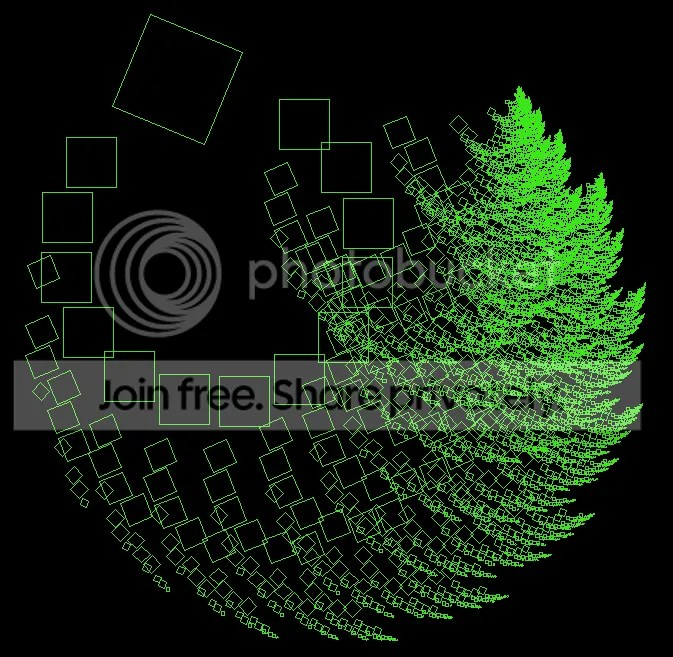# Bizarre Love Triangle Explained

Earlier I wrote about issues I have with Tuples and Functions. It was kind of long, rambling and tangential, so I decided to write about some concrete examples of what I’m talking about.

My first example pertains to the FunctionN implementations. For the linear transformation drawer, I tried writing a DSL with some sort of function application syntax that maps to underlying Scala methods (e.g. a Parser for “functionCall(arg0, arg1, … argX)”). I would like to simply write one method that takes any function and gives back a Parser for that function:

```def makeParser(functionX:SomeFunctionType):Parser[FunctionReturnType] = { ... }

val absParser = makeParser(math.abs _)
val powParser = makeParser(math.pow _)
```

What do I put in for SomeFunctionType? Nothing makes sense. Instead, I have to write makeParser1Arg, makeParser2Arg, etc. Code repetition should be avoided – but in this case, there’s no way around it. Even the Scala core library “solves” this problem through code repetition (that is, Scala has a TON of copypasta in FunctionN and TupleN).

As a second example, I wrote a simple grapher for the Lorenz Attractor that plotted each individual coordinate over time. I wanted to show each coordinate in a different color and also laid out below each other:So I wrote a method that looked like this:

```def draw(color:Int, y:Float, list:Seq[Float]) = {
translate(0, y);
stroke(color);
//handle draw...
}
```

I have a Seq[(Float, Float, Float)] representing the attractor’s states as it progresses through time. I can use unzip3 to get a (Seq[Float], Seq[Float], Seq[Float]) holding each individual coordinate as it progresses through time. Now, I want to call draw three times, passing each Seq as the third argument. I could hard-code it like so:

```//the original seq of the attractor's states
val states:Seq[(Float, Float, Float)] = ...

//Three Seqs of individual coordinates
val coords = states.unzip3;

draw(color(255, 0, 0), 0, coords._1);
draw(color(0, 255, 0), height/3f, coords._2);
draw(color(0, 0, 255), height*2/3f, coords._3);
```

The code repetition is pretty blatant. What if I wanted to draw 10 different lines? What about 100? Instead of explicitly calling draw with different values, I’d like to create two other containers – be them lists, tuples, whatever – holding the corresponding colors and y coordinates to be passed to the draw method.

```val colors = List(color(255, 0, 0), color(0, 255, 0), color(0, 0, 255));
val ys     = List(0f, height/3f, height*2/3f);
```

FunctionN’s have a .tupled method to let you pass a single TupleN to the function, letting you treat the arguments to pass to a Function as one object. All I have to do now is combine the three coords, colors, and ys Seqs into one Seq of Tuple3s, with each Tuple3 holding the corresponding arguments to pass to draw.

```//combine colors, ys, and coords somehow
val args:Seq[(Int, Float, Seq[Float])] = ...

args foreach (draw _).tupled //pass each set of args to the draw method
```

How do I do it? The answer is: very ugily (like, ugly-ly?). At the very least, you have to convert the coords from a Tuple3 of Seq[Float]s into a Seq of Seq[Float]s. There is no pretty way to do this. You can explicitly code it:

```//ewwwww
val coordsList = Seq(coords._1, coords._2, coords._3);
```

Or you can use the productIterator method of Tuple3, and then cast to an iterator of Seq[Float]s:

```//oh my jesus
val coordsList = coords.productIterator.asInstanceOf[Iterator[Seq[Float]]];
```

The first option isn’t extensible (if you want to plot a 5-dimensional attractor you’ll need to go back and explicitly change the code); the second option isn’t type-safe (if coords isn’t actually ALL Seq[Float]s, this won’t even exception until we try to actually explicitly grab one of the elements, at god knows what place).

But lets say we’ve gotten beyond that. Now we want to create the args sequence. We basically want the inverse of unzip3, taking in three seq arguments (one of them can be the caller) and outputting one Seq of Tuple3s. Seq has a “zip” method that does very, very close to what we want; Seq.zip only takes one argument and outputs a Seq of Tuple2s. But it’s not good enough. You can’t chain a zip with another zip, because that still only creates a Tuple2.

```//Seq[(Int, Float)]
val args2 = colors.zip(ys)

//Seq[((Int, Float), Seq[Float])]
val almost = args2.zip(coordsList)
```

Here are some solutions I’ve ended up with:

```//iterate through the index
val args = for(i &lt;- 0 until colors.length) yield (colors(i), ys(i), coordsList(i))

//same thing only slower! wooooo!
val args = colors.zipWithIndex.map { case (c, i) =&gt; (c, ys(i), coordsList(i)) }

//actually not terrible
val args = almost.map{ case ((a, b), c) =&gt; (a, b, c) }
```

# Parser Combinators are the shit!

The name is daunting, but parser combinators are actually pretty straightforward once you get the hang of it (as is the case with most programming :P). Scala has a parser combinator library built right in; I myself followed the chapter on Parser Combinators in Programming in Scala 2nd Edition (PiS2E). I won’t go into details about how such combinators work or write a tutorial; there are enough of them out there. It is sufficient to say that you can build a basic arithmetic calculator (with +, -, *, /, and parentheses) using THREE LINES (ok, six counting declarations). If that doesn’t whet your appetite, then your stomach is probably made of rubber… There’s probably a better pun in there somewhere, but I’ll leave that as an exercise for the reader :P The following code is taken directly from PiS2E:

```import scala.util.parsing.combinator._
class Arith extends JavaTokenParsers {
def expr: Parser[Any] = term~rep("+"~term | ""~term)
def term: Parser[Any] = factor~rep("*"~factor | "/"~factor)
def factor: Parser[Any] = floatingPointNumber | "("~expr~")"
}
```

All you need aside from that is to actually call the “parseAll” method with input, and then magic happens:

```println(parseAll(expr, args(0)))
input: 2 * (3 + 7)
[1.12] parsed: ((2~List((*~(((~((3~List())~List((+
~(7~List())))))~)))))~List())
```

The formatting isn’t particularly pleasing to look at but it’s apparent that the structure is in there. It’s not much more complicated to adapt the code to actually spit out a number, add in constants, or parse variables. It’s the shit.

I used parser combinators on a sketch about a week ago to let users type in linear transformations. I’ve been on a fractal binge lately, and I was reading about IFSes and decided to implement a simple IFS drawer for a sketch. The algorithm is pretty straightforward:

```  /*
a) you have a set of geometric objects (points, lines, polygons, curves, etc) (named S)
b) you have a set of transformations (rotate, translate, scale, shear, etc) (named T)
c) you apply each transformation to each object in that set, which gives you the set of transformed objects S' (giving you num(S)*num(T) new objects)
d) Use S' as the set S in a)
*/
def iterated(): Set[Geometry] = {
var Sprime: Set[Geometry] = Set()
S.foreach(g => T.foreach(t => Sprime += g.transformed(t)))
return Sprime;
}

def iterate() { //Move the iteration along.
S = iterated();
}
```

Writing a combinator to parse matrix inputs is pretty straightforward: you have basic transformations (translate, scale, rotate, etc.), and then you may combine them, usually with matrix multiplication (although I also implemented by-element summation to see what would happen). After some wiring and judicious ^^ usage (the ^^ method transforms raw combinator output types into types you want), some nice looking figures start popping up:At this point the only shape you start off with is a square; originally I was going to leave it at that but coming back to the sketch yesterday, I decided to add drawing capabilities. You can see the sketch here.I’m using controlP5 for the GUI, which works great for Processing but doesn’t have a comprehensive focus system so one has to write methods checking whether the user wants to draw things or if they’re just working the controls. controlP5 is also finnicky with regards to Proguard since the font it uses is stored in a couple of gif files within the “controlp5” package, so I decided to skip obfuscation for this jar; the resulting file is ~550kb, which still isn’t bad.

I wanted to create a three-dimensional view that kept track of the “age” of shapes and placed them on the Z axis according to age; that might be a future feature. All in all, I’m very pleased with how easy and quick it was to do what I was looking for in Scala, especially in regards to writing a grammar for linear transformations. It’s one of the reasons why I love Scala as a language; it’s like everything you ever wanted but didn’t have in Java. And so. Much. Less. Boilerplate. I’m not sure if I even USE plates anymore!